Number Theory and Public Key Cryptography

## Introduction to Number Theory

### Modular Arithmetic

• modular arithmetic is 'clock arithmetic'
• a congruence a = b mod n says when divided by n that a and b have the same remainder
• 100 = 34 mod 11
• usually have 0<=b<=n-1
• -12mod7 = -5mod7 = 2mod7 = 9mod7
• b is called the residue of a mod n
• can do arithmetic with integers modulo n with all results between 0 and n
addition
a+b mod n
subtraction
a-b mod n = a+(-b) mod n
multiplication
a.b mod n
• derived from repeated addition
• can get a.b=0 where neither a,b=0
• eg 2.5 mod 10
division
a/b mod n
• is multiplication by inverse of b: a/b = a.b-1 mod n
• if n is prime b-1 mod n exists s.t b.b-1 = 1 mod n
• eg 2.3=1 mod 5 hence 4/2=4.3=2 mod 5
• integers modulo n with addition and multiplication form a commutative ring with the laws of
Associativity
(a+b)+c = a+(b+c) mod n
Commutativity
a+b = b+a mod n
Distributivity
(a+b).c = (a.c)+(b.c) mod n
• also can chose whether to do an operation and then reduce modulo n, or reduce then do the operation, since reduction is a homomorphism from the ring of integers to the ring of integers modulo n
• a+/-b mod n = [a mod n +/- b mod n] mod n
• (the above laws also hold for multiplication)
• if n is constrained to be a prime number p then this forms a Galois Field modulo p denoted GF(p) and all the normal laws associated with integer arithmetic work

### Exponentiation in GF(p)

• many encryption algorithms use exponentiation - raising a number a (base) to some power b (exponent) mod p
• b = ae mod p
• exponentiation is basically repeated multiplication, which take s O(n) multiples for a number n
• a better method is the square and multiply algorithm
```  let base = a, result =1
for each bit ei (LSB to MSB) of exponent
if ei=0 then
square base mod p
if ei=1 then
multiply result by base mod p
square base mod p (except for MSB)
required ae is result
```
• only takes O(log2 n) multiples for a number n

see Sebbery p9 Fig2.1 + example

### Discrete Logarithms in GF(p)

• the inverse problem to exponentiation is that of finding the discrete logarithm of a number modulo p
• find x where ax = b mod p

Seberry examples p10

• whilst exponentiation is relatively easy, finding discrete logarithms is generally a hard problem, with no easy way
• in this problem, we can show that if p is prime, then there always exists an a such that there is always a discrete logarithm for any b!=0
• successive powers of a "generate" the group mod p
• such an a is called a primitive root and these are also relatively hard to find

### Greatest Common Divisor

• the greatest common divisor (a,b) of a and b is the largest number that divides evenly into both a and b
• Euclid's Algorithm is used to find the Greatest Common Divisor (GCD) of two numbers a and n, a<n
• use fact if a and b have divisor d so does a-b, a-2b
```GCD (a,n) is given by:
let g0=n
g1=a
gi+1 = gi-1 mod gi
when gi=0 then (a,n) = gi-1
```
eg find (56,98)
```   g0=98
g1=56
g2 = 98 mod 56 = 42
g3 = 56 mod 42 = 14
g4 = 42 mod 14 = 0
```
hence (56,98)=14

see Seberry Fig 2.2 p11 GCD alg 

### Inverses and Euclid's Extended GCD Routine

• unlike normal integer arithmetic, sometimes a number in modular arithmetic has a unique inverse
• a-1 is inverse of a mod n if a.a-1 = 1 mod n
• where a,x in {0,n-1}
• eg 3.7 = 1 mod 10
• if (a,n)=1 then the inverse always exists
• can extend Euclid's Algorithm to find Inverse by keeping track of gi = ui.n + vi.a
• Extended Euclid's (or Binary GCD) Algorithm to find Inverse of a number a mod n (where (a,n)=1) is:
```  Inverse(a,n) is given by:
g0=n  u0=1  v0=0
g1=a  u1=0  v1=1
let
y = gi-1 div gi
gi+1 = gi-1 - y.gi = gi-1 mod gi
ui+1 = ui-1 - y.ui
vi+1 = vi-1 - y.vi
when gi=0 then Inverse(a,n) = vi-1
```
Example

eg: want to find Inverse(3,460):

```
```
```i          y          g          u          v
0          -          460        1          0
1          -          3          0          1
2          153        1          1          -153
3          3          0          -3         460

```
```
hence Inverse(3,460) = -153 = 307 mod 460
```

see Seberry Fig 2.3 p14 Inverse alg 

### Euler Totient Function [[phi]](n)

• if consider arithmetic modulo n, then a reduced set of residues is a subset of the complete set of residues modulo n which are relatively prime to n
• eg for n=10,
• the complete set of residues is {0,1,2,3,4,5,6,7,8,9}
• the reduced set of residues is {1,3,7,9}
• the number of elements in the reduced set of residues is called the Euler Totient function [[phi]](n)
• there is no single formula for [[phi]](n) but for various cases count how many elements are excluded:
```	p (p prime)	[[phi]](p) =p-1
pr (p prime)	[[phi]](p) =pr-1(p-1)
p.q (p,q prime)	[[phi]](p.q) =(p-1)(q-1)
```

see Seberry Table 2.1 p13

• several important results based on [[phi]](n) are:
• Theorem (Euler's Generalization)
• let gcd(a,n)=1 then
• a[[phi]](n) mod n = 1
• Fermat's Theorem
• let p be a prime and gcd(a,p)=1 then
• ap-1 mod p = 1
• Algorithms to find Inverses a-1 mod n
1. search 1,...,n-1 until an a-1 is found with a.a-1 mod n
2. if [[phi]](n) is known, then from Euler's Generalization
• a-1 = a[[phi]](n)-1 mod n
3. otherwise use Extended Euclid's algorithm for inverse

### Computing with Polynomials in GF(qn)

• have seen arithmetic modulo a prime number GF(p)
• also can do arithmetic modulo q over polynomials of degree n, which also form a Galois Field GF(qn)
• its elements are polynomials of degree (n-1) or lower
• a(x)=an-1xn-1+an-2xn-2+...+a1x+a0
• have residues for polynomials just as for integers
• p(x)=q(x)d(x)+r(x)
• and this is unique if deg[r(x)]<deg[d(x)]
• if r(x)=0, then d(x) divides p(x), or is a factor of p(x)
• addition in GF(qn) just involves summing equivalent terms in the polynomial modulo q (XOR if q=2)
• a(x)+b(x)=(an-1+bn-1)xn-1+...+(a1+b1)x+(a0+b0)

### Multiplication with Polynomials in GF(qn)

• multiplication in GF(qn) involves 
• multiplying the two polynomials together (cf longhand multiplication; here use shifts & XORs if q=2)
• then finding the residue modulo a given irreducible polynomial of degree n
• an irreducible polynomial d(x) is a 'prime' polynomial, it has no polynomial divisors other than itself and 1
• modulo reduction of p(x) consists of finding some r(x) st: p(x)=q(x)d(x)+r(x)
• nb. in GF(2n) with d(x)=x3+x+1 can do simply by replacing x3 with x+1
• eg in GF(23) there are 8 elements:
• 0, 1, x, x+1, x2, x2+1, x2+x, x2+x+1
• with irreducible polynomial d(x)=x3+x+1* arithmetic in this field can be summarised as:

Seberry Table 2.3 p20

• can adapt GCD, Inverse, and CRT algorithms for GF(qn)
• [[phi]](p(x)) = 2n-1 since every poly except 0 is relatively prime to p(x)
• arithmetic in GF(qn) can be much faster than integer arithmetic, especially if the irreducible polynomial is carefully chosen
• eg a fast implementation of GF(2127) exists
• has both advantages and disadvantages for cryptography, calculations are faster, as are methods for breaking

## Complexity Theory - A Potted Intro

• Complexity theory - study of how hard a problem is to solve in general
• allows classification of types of problems
• some problems intrinsically harder than others, eg
• multiplying numbers O(n^(2))
• multiplying matrices O(n^(2)(2n-1))
• solving crossword O(26^(n))
• recognizing primes O(n^(log log n))
• deal with worst case complexity
• may on average be easier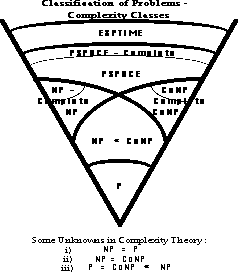### Complexity Theory - Some Terminolgy

• an instance of a problem is a particular case of a general problem
• the input length of a problem is the number n of symbols used to characterize a particular instance of it
• the order of a function f(n) - is some O(g(n)) of some function g(n) s.t.
• f(n)<=c.|g(n)|, for all n>=0, for some c
• a polynomial time algorithm (P) is one which solves any instance of a particular problem in a length of time O(p(n)), where p is some polynomial on input length
• an exponential time algorithm (E) - is one whose solution time is not so bounded
• a non-deterministic polynomial time algorithm (NP) - is one for which any guess at the solution of an instance of the problem may be checked for validity in polynomial time
• NP-complete problems - are a subclass of NP problems for which it is known that if any such problem has a polynomial time solution, then all NP problems have polynomial solutions. These are thus the hardest NP problems
• Co-NP problems - are the complements of NP problems, to prove a guess at a solution of a Co-NP problem may well require an exhaustive search of the solution space

## Public-Key Ciphers

• traditional secret key cryptography uses a single key shared by both sender and receiver
• if this key is disclosed communications are compromised
• also does not protect sender from receiver forging a message & claiming is sent by sender, parties are equal
• public-key (or two-key) cryptography involves the use of two keys:
• a public-key, which may be known by anybody, and can be used to encrypt messages, and verify signatures
• a private-key, known only to the recipient, used to decrypt messages, and sign (create) signatures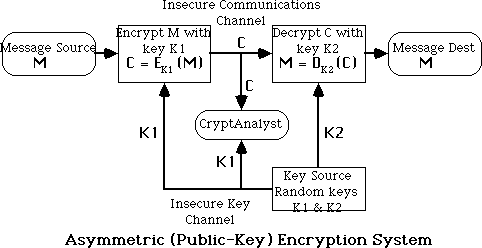• the public-key is easily computed from the private key and other information about the cipher (a polynomial time (P-time) problem)
• however, knowing the public-key and public description of the cipher, it is still computationally infeasible to compute the private key (an NP-time problem)
• thus the public-key may be distributed to anyone wishing to communicate securly with its owner (although secure distribution of the public-key is a non-trivial problem - the key distribution problem)
• have three important classes of public-key algorithms:
• Public-Key Distribution Schemes (PKDS) - where the scheme is used to securly exchange a single piece of information (whose value depends on the two parties, but cannot be set).
• This value is normally used as a session key for a private-key scheme
• Signature Schemes - used to create a digital signature only, where the private-key signs (create) signatures, and the public-key verifies signatures
• Public Key Schemes (PKS) - used for encryption, where the public-key encrypts messages, and the private-key decrypts messages.
• Any public-key scheme can be used as a PKDS, just by selecting a message which is the required session key
• Many public-key schemes are also signature schemes (provided encryption & decryption can be done in either order)
Digital Signatures and Signature Schemes
• where the private-key signs (create) signatures, and the public-key verifies signatures
• only the owner can create the digital signature, hence it can be used to verify who created a message
• generally don't sign the whole message (doubling the size of information exchanged), but just a digest or hash of the message,
• a hash function takes the message, and produces a fixed size (typically 64 to 512 bits) value dependent on the message
• it must be hard to create another message with the same hash value (otherwise some forgeries are possible)
• developing good hash functions is another non-trivial problem

### Diffie-Hellman Public-Key Distribution Scheme

• first public-key type scheme proposed was a PKDS by Diffie & Hellman in 1976:
W Diffie, M E Hellman, "New directions in Cryptography", IEEE Trans. Information Theory, IT-22, pp644-654, Nov 1976
• an excellent overview of cryptography at this time is:
W Diffie, M E Hellman, "Privacy and Authentication: An Introduction to Cryptography", Proc. of the IEEE, Vol 67 No 3, pp 397-427, Mar 1979
• it is a public-key distribution scheme
• it cannot be used to exchange an arbitrary message
• only a key, whose value depends on the participants (and their private and public key information)
• is based on exponentiation in a finite (Galois) field, either over integers modulo a prime, or a polynomial field
• nb exponentiation takes O((log n)3) operations
• its security relies on the difficulty of computing logarithms in these fields
• nb discrete logarithms takes O(e log n log log n) operations
• Diffie-Hellman PKDS works as follows:
• two people A & B, who wish to exchange some key over an insecure communications channel can:
• select a large prime p (~200 digit), and
• [[alpha]] a primitive element mod p
• A has a secret number xA
• B has a secret number xB
• A and B compute yA and yB respectively, which are then made public
• yA = [[alpha]]xA mod p yB = [[alpha]]xB mod p
• the key is then calculated as
• KAB = [[alpha]]xA.xB mod p
• = yAxB mod p (which B can compute)
• = yBxA mod p (which A can compute)
• and may then be used in a private-key cipher to secure communications between A and B
• nb: if the same two people subsequently wish to communicate, they will have the same key as before, unless they change their public-key (usually not often)

### RSA Public-Key Cryptosystem

• best known and widely regarded as most practical public-key scheme was proposed by Rivest, Shamir & Adleman in 1977:
R L Rivest, A Shamir, L Adleman, "On Digital Signatures and Public Key Cryptosystems", Communications of the ACM, vol 21 no 2, pp120-126, Feb 1978
• it is a public-key scheme which may be used for encrypting messages, exchanging keys, and creating digital signatures
• is based on exponentiation in a finite (Galois) field over integers modulo a prime
• nb exponentiation takes O((log n)3) operations
• its security relies on the difficulty of calculating factors of large numbers
• nb factorization takes O(e log n log log n) operations
• (same as for discrete logarithms)
• the algorithm is patented in North America (although algorithms cannot be patented elsewhere in the world)
• this is a source of legal difficulties in using the scheme
• RSA is a public key encryption algorithm based on exponentiation using modular arithmetic
• to use the scheme, first generate keys:
• Key-Generation by each user consists of:
• selecting two large primes at random (~100 digit), p, q
• calculating the system modulus R=p.q p, q primes
• selecting at random the encryption key e,
• e < R, gcd(e, F(R)) = 1
• solving the congruence to find the decryption key d,
• e.d [[equivalence]] 1 mod [[phi]](R) 0 <= d <= R
• publishing the public encryption key: K1={e,R}
• securing the private decryption key: K2={d,p,q}
• Encryption of a message M to obtain ciphertext C is:
• C = Me mod R 0 <= d <= R
• Decryption of a ciphertext C to recover the message M is:
• M = Cd = Me.d = M1+n.[[phi]](R) = M mod R
• the RSA system is based on the following result:
if R = pq where p, q are distinct large primes then
```	X [[phi]](R) = 1 mod R
for all x not divisible by p or q
and [[Phi]](R) = (p-1)(q-1)
```

#### RSA Example

• usually the encryption key e is a small number, which must be relatively prime to [[phi]](R) (ie GCD(e, [[phi]](R)) = 1)
• typically e may be the same for all users (provided certain precautions are taken), 3 is suggested
• the decryption key d is found by solving the congruence:
```		e.d [[equivalence]] 1 mod [[phi]](R),	0 <= d <= R,
```
• an extended Euclid's GCD or Binary GCD calculation is done to do this.
```	given e=3, R=11*47=517, [[phi]](R)=10*46=460
then d=Inverse(3,460) by Euclid's alg:
i    y    g    u    v
0    -  460    1    0
1    -    3    0    1
2  153    1    1 -153
3    3    0   -3  460
ie:	d = -153, or 307 mod 517
```
• a sample RSA encryption/decryption calculation is:
```	M = 26
C = 263 mod 517 = 515
M = 515307 mod 517 = 26
```

#### Security of RSA

• The security of the RSA scheme rests on the difficulty of factoring the modulus of the scheme R
• best known factorization algorithm (Brent-Pollard) takes: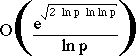operations on number R whose largest prime factor is p

```Decimal Digits in R                          #Bit Operations to Factor R
20                                         7200
40                                         3.11e+06
60                                         4.63e+08
80                                         3.72e+10
100                                          1.97e+12
120                                          7.69e+13
140                                          2.35e+15
160                                          5.92e+16
180                                          1.26e+18
200                                          2.36e+19

```
• This leads to R having a length of 200 digits (or 600 bits) given that modern computers perform 1-100 MIPS the above can be divided by 106 to get a time in seconds
• nb: currently 1e+14 operations is regarded as a limit for computational feasability and there are 3e+13 usec/year
• but most (all!!) computers can't directly handle numbers larger than 32-bits (64-bits on the very newest)
• hence need to use multiple precision arithmetic libraries to handle numbers this large

#### Multi-Precision Arithmetic

• involves libraries of functions that work on multiword (multiple precision) numbers
• classic references are in Knuth vol 2 - "Seminumerical Algorithms"
• multiplication digit by digit
• do exponentiation using square and multiply
• are a number of well known multiple precision libraries available - so don't reinvent the wheel!!!!
• can use special tricks when doing modulo arithmetic, especially with the modulo reductions

#### Faster Modulo Reduction

* Chivers (1984) noted a fast way of performing modulo reductions whilst doing multi-precision arithmetic calcs

Given an integer A of n characters (a0, ... , an-1) of base b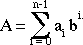then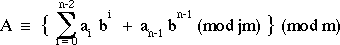ie: this implies that the MSD of a number can be removed and its remainder mod m added to the remaining digits will result in a number that is congruent mod m to the original.

* Chivers algorithm for reducing a number is thus:

1. Construct an array R = (bd, 2.bd, ... , (b-1).bd)(mod m)

2. FOR i = n-1 to d do

WHILE A[i] != 0 do

j = A[i];

A[i] = 0;

A = A + bi-d.R[j];

END WHILE

END FOR

where A[i] is the ith character of number A

R[j] is the jth integer residue from the array R

n is the number of symbols in A

d is the number of symbols in the modulus

#### Speeding up RSA - Alternate Multiplication Techniques

• conventional multiplication takes O(n2) bit operations, faster techniques include:
• the Schonhage-Strassen Integer Multiplication Algorithm:
• breaks each integer into blocks, and uses them as coefficients of a polynomial
• evaluates these polynomials at suitable points, & multiplies the resultant values
• interpolates these values to form the coefficients of the product polynomial
• combines the coefficients to form the product of the original integer
• the Discrete Fourier Transform, and the Convolution Theorem are used to speed up the interpolation stage
• can multiply in O(n log n) bit operations
• the use of specialized hardware because:
• conventional arithmetic units don't scale up, due to carry propogation delays
• so can use serial-parallel carry-save, or delayed carry-save techniques with O(n) gates to multiply in O(n) bit operations,
• or can use parallel-parallel techniques with O(n2) gates to multiply in O(log n) bit operations

#### RSA and the Chinese Remainder Theorem

• a significant improvement in decryption speed for RSA can be obtained by using the Chinese Remainder theorem to work modulo p and q respectively
• since p,q are only half the size of R=p.q and thus the arithmetic is much faster
• CRT is used in RSA by creating two equations from the decryption calculation:
```	M = Cd mod R
```
as follows:
```	M1 = M mod p = (C mod p)d mod (p-1)
M2 = M mod q = (C mod q)d mod (q-1)
```
then the pair of equations
```	M = M1 mod p	M = M2 mod q
```
has a unique solution by the CRT, given by:
```	M = [((M2 +q - M1)u mod q] p + M1
```
where
```		p.u mod q = 1
```

#### Primality Testing and RSA

• The first stage of key-generation for RSA involves finding two large primes p, q
• Because of the size of numbers used, must find primes by trial and error
• Modern primality tests utilize properties of primes eg:
• an-1 = 1 mod n where GCD(a,n)=1
• all primes numbers 'n' will satisfy this equation
• some composite numbers will also satisfy the equation, and are called pseudo-primes.
• Most modern tests guess at a prime number 'n', then take a large number (eg 100) of numbers 'a', and apply this test to each. If it fails the number is composite, otherwise it is is probably prime.
• There are a number of stronger tests which will accept fewer composites as prime than the above test. eg: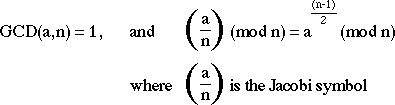#### RSA Implementation in Practice

• Software implementations
• generally perform at 1-10 bits/second on block sizes of 256-512 bits
• two main types of implementations:
• - on micros as part of a key exchange mechanism in a hybrid scheme
• - on larger machines as components of a secure mail system
• Harware Implementations
• generally perform 100-10000 bits/sec on blocks sizes of 256-512 bits
• all known implementations are large bit length conventional ALU units

### El Gamal

• a variant of the Diffie-Hellman key distribution scheme, allowing secure exchange of messages
• published in 1985 by ElGamal in
T. ElGamal, "A Public Key Cryptosystem and a Signature Scheme Based on Discrete Logarithms", IEEE Trans. Information Theory, vol IT-31(4), pp469-472, July 1985.
• like Diffie-Hellman its security depends on the difficulty of factoring logarithms
• Key Generation
• select a large prime p (~200 digit), and
• [[alpha]] a primitive element mod p
• A has a secret number xA
• B has a secret number xB
• A and B compute yA and yB respectively, which are then made public
• yA = [[alpha]]xA mod p
• yB = [[alpha]]xB mod p
• to encrypt a message M into ciphertext C,
• selects a random number k, 0 <= k <= p-1
• computes the message key K
• K = yBk mod p
• computes the ciphertext pair: C = {c1,c2}
• C1 = [[alpha]]k mod p C2 = K.M mod p
• to decrypt the message
• extracts the message key K
• K = C1xB mod p = [[alpha]]k.xB mod p
• extracts M by solving for M in the following equation:
• C2 = K.M mod p

### Other Public-Key Schemes

• a number of other public-key schemes have been proposed, some of the better known being:
• Knapsack based schemes
• McEleice's Error Correcting Code based schems
• ALL of these schemes have been broken
• the only currently known secure public key schemes are those based on exponentiation
(all of which are patented in North America)

• it has proved to be very very difficult to develop secure public key schemes
• this in part is why they have not been adopted faster, as their theorectical advantages might have suggested

 examples: 7^5 -> a=7, e={101}
 more examples: GCD(84,60)=12; GCD(323,238)=17; GCD(323,299)=1;
more examples: Inv(299,323)=148 show trace of g=u.n+v.a
 show examples: eg [[phi]] of 3, 3.3, 3.5
 example: a=x^2+1, find a^2 mod (x^3+x+1)=x^2+x+1 showing modulo reduction process
 follow with examples of multiple precision arithmetic
[CSC Info]
Lawrie.Brown@adfa.oz.au / 24-Apr-96